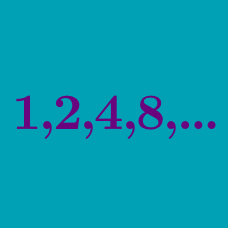Algebra

# Geometric Mean

What is the geometric mean of 5 and 45?

What is the geometric mean of the following numbers:

$63, 147, 27$

There is a cuboid with dimensions $10 \times a \times b.$ If $10, a, b$ form a geometric progression and the volume of the cuboid is $1000,$ what is the value of $a?$

What is the geometric mean of ${4}^1, {4}^2, \cdots, {4}^n?$

What is the geometric mean of the following numbers:

$16, 12, 9 ?$

×